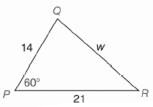Chapter 11.CR, Problem 12CR### Elementary Geometry for College St...

6th Edition
Daniel C. Alexander + 1 other
ISBN: 9781285195698

#### Solutions

Chapter
Section### Elementary Geometry for College St...

6th Edition
Daniel C. Alexander + 1 other
ISBN: 9781285195698
Textbook Problem
1 views

# In Exercises 9 to 12, use the Law of S i n e s or the Law of C o s i n e s to find the indicated length of side or angle measure. Angle measures should be found to the nearest degree; distances should be found to the nearest tenth of a unit.To determine

To find:

The indicated length of side or angle measure using the law of sines or the Law of cosines.

Explanation

Procedure used:

In any triangle ABC such that

AB=c,

BC=a,

CA=b,

mA=α,

mB=β, and

mC=γ.

The Law of cosines is given by

a2=b2+c2-2bccosα

b2=c2+a2-2cacosβ

c2=a2+b2-2abcosγ

The Law of sines is given by

asinα=bsinβ=csinγ

Calculation:

Given:

### Still sussing out bartleby?

Check out a sample textbook solution.

See a sample solution

#### The Solution to Your Study Problems

Bartleby provides explanations to thousands of textbook problems written by our experts, many with advanced degrees!

Get Started

#### Find more solutions based on key concepts